Do you like the article?
Share it with others —
Use new possibilities of MetaTrader 5

#### Similar articles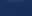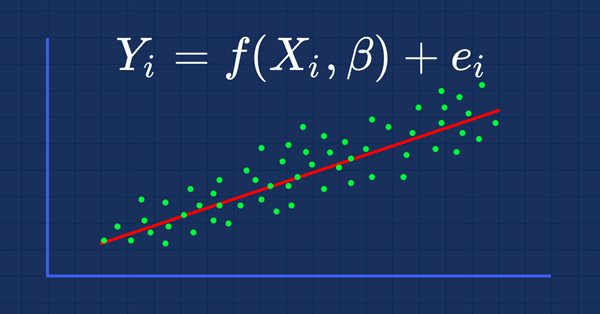# MQL5 Wizard techniques you should know (Part 01): Regression Analysis

MetaTrader 5Tester | 21 June 2022, 15:455 2501### 1. Introduction

MQL5 wizard allows the rapid construction and deployment of expert advisors by having most of the menial aspects of trading pre-coded in the MQL5 library. This allows traders to focus on their custom aspects of their trading such as special entry and exit conditions. Included in the library are some entry and exit signal classes like signals of 'Accelerator Oscillator' indicator, or signals of 'Adaptive Moving Average' indicator and many others. Besides being based on lagging indicators for most traders they may not be convertible to successful strategies. This is why the ability to create your own custom signal is essential. For this article we will explore how this can be done with regression analysis.

### 2. Creating the class

2.1 Regression analysis, per Wikipedia, is a set of statistical processes for estimating the relationships between a dependent variable and one or more independent variables. It can be useful to a trader’s expert signal since price data is a time series. This, therefore, allows one to test for the ability of prior prices and price changes to influence future prices and price changes. Regression analysis can be represented by the equation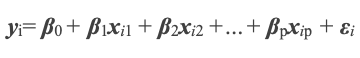Where y the dependent and therefore predicted variable depends on prior x values each with their own coefficient β, and an error ε. We can think of values and the value to be previous and projected price levels respectively. Besides working with price levels, price changes can also be examined in similar fashion. The unknown y is dependent on xs, βs, and ε. Of these though only the xs and β0 (the y-intercept) are known. The y-intercept is known because it is the price immediately before  x i 1. We therefore need to find the respective βs for each x and then the ε Because each x i 1 was a y I at the prior time point of the time series, we can solve for the β values using simultaneous equations. If the next change in price is dependent on only two prior changes, for example, our current equation could be: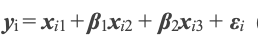And the previous equations would be: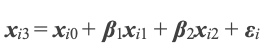Since we estimate error ε separately, we can solve the two simultaneous equations for the β values. The numbering of the x values in the Wikipedia formula is not in the MQL5 'series' format meaning the highest numbered x is the most recent. I have thus renumbered the x values in the above 2 equations to show how they can be simultaneous. Again we start with the y-intercepts xi1 and xi0 to represent β0 in equation 1. The solving of simultaneous equations is better handled with matrices for efficiency. The tools for this are in the MQL5 Library.

2.2  MQL5 library has an extensive collection of classes on statistics, and common algorithms that clearly negate the need for one to have to code them from scratch. Its code is also open to the public meaning it can be independently checked. For our purposes we’ll use the ‘RMatrixSolve’ function, under class ‘CDenseSolver’ in the 'solvers.mqh' file. At the heart of this function is the use of matrix LU decomposition to quickly and efficiently solve for β values. Articles have been written on this in the MetaQuotes archive and Wikipedia also has an explanation here

Before we delve into solving for β values it would be helpful to look at how the ‘CExpertSignal’ class is structured as it is the basis for our class. In almost all expert signal classes that can be assembled in the wizard, there is a ‘LongCondition’ function and a ‘ShortCondition’ function. As you would expect, the two return a value that sets whether you should go long or short respectively. This value needs to be an integer in the range of 0 to 100 in order to map with the wizard’s input parameters of ‘Signal_ThresholdOpen’ and ‘Signal_ThresholdClose’. Typically, when trading you want your conditions for closing a position to be less conservative than your conditions for opening. This means the threshold for opening will be higher than the threshold for closing. In developing our signal therefore, we are going to have input parameters for computing the close threshold and separate but similar input parameters for the opening threshold. The selection of inputs to use when computing a condition will be determined by whether or not we have open positions. If we have open positions we will use the close parameters. If no positions are present we will use open parameters. The listing of our expert signal class interface that shows these two sets of parameters is below.

```//+------------------------------------------------------------------+
//|                                                                  |
//+------------------------------------------------------------------+
class CSignalDUAL_RA : public CExpertSignal
{
protected:
CiMA              m_h_ma;             // highs MA handle
CiMA              m_l_ma;             // lows MA handle
CiATR             m_ATR;
int               m_size;
double            m_open_determination,m_close_determination;
int               m_open_collinearity,m_open_data,m_open_error;
int               m_close_collinearity,m_close_data,m_close_error;
public:
CSignalDUAL_RA();
~CSignalDUAL_RA();
//--- methods of setting adjustable parameters

//--- PARAMETER FOR SETTING THE NUMBER OF INDEPENDENT VARIABLES
void              Size(int value)                  { m_size=value;                  }

//--- PARAMETERS FOR SETTING THE OPEN 'THRESHOLD' FOR THE EXPERTSIGNAL CLASS
void              OpenCollinearity(int value)      { m_open_collinearity=value;     }
void              OpenDetermination(double value)  { m_open_determination=value;    }
void              OpenError(int value)             { m_open_error=value;            }
void              OpenData(int value)              { m_open_data=value;             }

//--- PARAMETERS FOR SETTING THE CLOSE 'THRESHOLD' FOR THE EXPERTSIGNAL CLASS
void              CloseCollinearity(int value)     { m_close_collinearity=value;    }
void              CloseDetermination(double value) { m_close_determination=value;   }
void              CloseError(int value)            { m_close_error=value;           }
void              CloseData(int value)             { m_close_data=value;            }

//--- method of verification of settings
virtual bool      ValidationSettings(void);
//--- method of creating the indicator and timeseries
virtual bool      InitIndicators(CIndicators *indicators);
//--- methods for detection of levels of entering the market
virtual bool      OpenLongParams(double &price,double &sl,double &tp,datetime &expiration);
virtual bool      OpenShortParams(double &price,double &sl,double &tp,datetime &expiration);
//--- methods of checking if the market models are formed
virtual int       LongCondition(void);
virtual int       ShortCondition(void);
protected:
//--- method of initialization of the oscillator
bool              InitRA(CIndicators *indicators);
//--- methods of getting data
int               CheckDetermination(int ind,bool close);
double            CheckCollinearity(int ind,bool close);
//
double            GetY(int ind,bool close);
double            GetE(int ind,bool close);

double            Data(int ind,bool close);
//
};```

Also, here is a listing of our ‘LongCondition’ and ‘ShortCondition’ functions.

```//+------------------------------------------------------------------+
//|                                                                  |
//+------------------------------------------------------------------+
int CSignalDUAL_RA::LongCondition(void)
{
int _check=CheckDetermination(0,PositionSelect(m_symbol.Name()));
if(_check>0){ return(_check); }

return(0);
}
//+------------------------------------------------------------------+
//|                                                                  |
//+------------------------------------------------------------------+
int CSignalDUAL_RA::ShortCondition(void)
{
int _check=CheckDetermination(0,PositionSelect(m_symbol.Name()));
if(_check<0){ return((int)fabs(_check)); }

return(0);
}```

To continue though, in order to solve for β values, we will use the ‘GetY’ function. This is listed below.

```//+------------------------------------------------------------------+
//|                                                                  |
//+------------------------------------------------------------------+
double CSignalDUAL_RA::GetY(int ind,bool close)
{
double _y=0.0;

CMatrixDouble _a;_a.Resize(m_size,m_size);
double _b[];ArrayResize(_b,m_size);ArrayInitialize(_b,0.0);

for(int r=0;r<m_size;r++)
{
_b[r]=Data(r,close);

for(int c=0;c<m_size;c++)
{
_a[r].Set(c,Data(r+c+1, close));
}
}

int _info=0;
CDenseSolver _S;
CDenseSolverReport _r;
double _x[];ArrayResize(_x,m_size);ArrayInitialize(_x,0.0);

_S.RMatrixSolve(_a,m_size,_b,_info,_r,_x);

for(int r=0;r<m_size;r++)
{
_y+=(Data(r,close)*_x[r]);
}
//---
return(_y);
}```

The ‘Data’ function referred to will switch between changes in the close price of the symbol being traded or changes in the moving average of the same close price. The option used will be defined by either the ‘m_open_data’ input parameter or the ‘m_close_data’ input parameter depending on whether we are computing the open threshold or the close threshold. The listing for selecting data is shown in the enumeration below.

```enum Edata
{
DATA_TREND=0,        // changes in moving average close
DATA_RANGE=1         // changes in close
};```

And the ‘Data’ function that selects this is listed below.

```//+------------------------------------------------------------------+
//|                                                                  |
//+------------------------------------------------------------------+
double CSignalDUAL_RA::Data(int ind,bool close)
{
if(!close)
{
if(Edata(m_open_data)==DATA_TREND)
{
m_h_ma.Refresh(-1);
return((m_l_ma.Main(StartIndex()+ind)-m_l_ma.Main(StartIndex()+ind+1))-(m_h_ma.Main(StartIndex()+ind)-m_h_ma.Main(StartIndex()+ind+1)));
}
else if(Edata(m_open_data)==DATA_RANGE)
{
return((Low(StartIndex()+ind)-Low(StartIndex()+ind+1))-(High(StartIndex()+ind)-High(StartIndex()+ind+1)));
}
}
else if(close)
{
if(Edata(m_close_data)==DATA_TREND)
{
m_h_ma.Refresh(-1);
return((m_l_ma.Main(StartIndex()+ind)-m_l_ma.Main(StartIndex()+ind+1))-(m_h_ma.Main(StartIndex()+ind)-m_h_ma.Main(StartIndex()+ind+1)));
}
else if(Edata(m_close_data)==DATA_RANGE)
{
return((Low(StartIndex()+ind)-Low(StartIndex()+ind+1))-(High(StartIndex()+ind)-High(StartIndex()+ind+1)));
}
}

return(0.0);
}```

Once we have the β values we can then proceed to estimate the error.

2.3  Standard Error according to Wikipedia can be estimated with the formula below.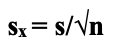With as the standard deviation and n the sample size, the error serves as a sobering reminder that not all projections no matter how diligent, will be 100% accurate all the time. We should always factor in and expect some error on our part. The standard deviation, shown in the formula, will be measured between our predicted values and the actual values. For comparison purposes we can also look at a raw error such as the last difference between our forecast and the actual. These two options can be selected from the enumeration below.

```enum Eerror
{
ERROR_LAST=0,        // use the last error
ERROR_STANDARD=1     // use standard error
}```

The ’GetE’ function will then return our error estimate depending on the input parameters ‘m_open_error’ or ‘m_close_error’ while using the formula above. This is listed below.

```//+------------------------------------------------------------------+
//|                                                                  |
//+------------------------------------------------------------------+
double CSignalDUAL_RA::GetE(int ind,bool close)
{
if(!close)
{
if(Eerror(m_open_error)==ERROR_STANDARD)
{
double _se=0.0;
for(int r=0;r<m_size;r++) { _se+=pow(Data(r,close)-GetY(r+1,close),2.0); }
_se=sqrt(_se/(m_size-1)); _se=_se/sqrt(m_size); return(_se);
}
else if(Eerror(m_open_error)==ERROR_LAST){ return(Data(ind,close)-GetY(ind+1,close)); }
}
else if(close)
{
if(Eerror(m_close_error)==ERROR_STANDARD)
{
double _se=0.0;
for(int r=0;r<m_size;r++){  _se+=pow(Data(r,close)-GetY(r+1,close),2.0); }
_se=sqrt(_se/(m_size-1)); _se=_se/sqrt(m_size); return(_se);
}
else if(Eerror(m_close_error)==ERROR_LAST){ return(Data(ind,close)-GetY(ind+1,close)); }
}
//---
return(Data(ind,close)-GetY(ind+1,close));
}```

Once again, the use of ‘m_open_error’ or ‘m_close_error’ will be determined by whether or not we have open positions. Once we have our error estimate we should be able to make a ball park prediction for y. Regression analysis however has a number of pitfalls. One such pitfall is the ability of the independent variables to be too similar and therefore over inflate the predicted value. This phenomenon is called collinearity and is worth checking for.

2.4  Collinearity which Wikipedia defines here, can be surmised as the occurrence of high intercorrelations among two or more independent variables in a multiple regression model as per Investopedia. It does not have a formula per se and is detected by the variance inflation factor (VIF). This factor is measured across all the independent variables (x) to help get a sense of how each of these variables is unique in predicting y. It is given by the formula below where R is the regression of each independent variable against the others.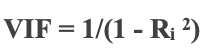For our purposes though, in taking account of collinearity, we will take the inverse of the spearman correlation between two recent data sets of independent variables and normalise it. Our data sets length will be set by the input parameter ‘m_size’ whose minimum length is 3. By normalization we will simply subtract it from two and invert the result. This normalized weight can then be multiplied either to the error estimate, or the predicted value, or both, or be unused. These options are listed in the enumeration below.

```enum Echeck
{
CHECK_Y=0,           // check for y only
CHECK_E=1,           // check for the error only
CHECK_ALL=2,         // check for both the y and the error
CHECK_NONE=-1        // do not use collinearity checks
};```

The choice of the applied weight is also set by either input parameter ‘m_open_collinearity’ or ‘m_close_collinearity’. Again, depending on if positions are open. The ‘CheckCollinearity’ listing is given below.

```//+------------------------------------------------------------------+
//|                                                                  |
//+------------------------------------------------------------------+
double CSignalDUAL_RA::CheckCollinearity(int ind,bool close)
{
double _check=0.0;
double _c=0.0,_array_1[],_array_2[],_r=0.0;
ArrayResize(_array_1,m_size);ArrayResize(_array_2,m_size);
ArrayInitialize(_array_1,0.0);ArrayInitialize(_array_2,0.0);
for(int s=0; s<m_size; s++)
{
_array_1[s]=Data(ind+s,close);
_array_2[s]=Data(m_size+ind+s,close);
}
_c=1.0/(2.0+fmin(-1.0,MathCorrelationSpearman(_array_1,_array_2,_r)));

double   _i=Data(m_size+ind,close),    //y intercept
_y=GetY(ind,close),           //product sum of x and its B coefficients
_e=GetE(ind,close);           //error

if(!close)
{
if(Echeck(m_open_collinearity)==CHECK_Y){ _check=_i+(_c*_y)+_e;          }
else if(Echeck(m_open_collinearity)==CHECK_E){ _check=_i+_y+(_c*_e);     }
else if(Echeck(m_open_collinearity)==CHECK_ALL){ _check=_i+(_c*(_y+_e)); }
else if(Echeck(m_open_collinearity)==CHECK_NONE){ _check=_i+(_y+_e);     }
}
else if(close)
{
if(Echeck(m_close_collinearity)==CHECK_Y){ _check=_i+(_c*_y)+_e;          }
else if(Echeck(m_close_collinearity)==CHECK_E){ _check=_i+_y+(_c*_e);     }
else if(Echeck(m_close_collinearity)==CHECK_ALL){ _check=_i+(_c*(_y+_e)); }
else if(Echeck(m_close_collinearity)==CHECK_NONE){ _check=_i+(_y+_e);     }
}

//---
return(_check);
}```

Besides checking for collinearity, there are times when regression analysis is not as predictive as it could be due to exogeneous changes in the market. To keep track of this, and measure the ability of our signal’s independent variables to influence our dependent variable (the forecast), we use the coefficient of determination.

2.5  Coefficient of determination is a statistical measurement that examines how differences in one variable can be explained by the difference in a second variable, when predicting the outcome of a given event as per Investopedia. Wikipedia also provides a more exhaustive definition and our formulae shown below are adopted from there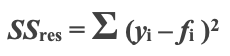The formula for sum of squares (with y be the actual value and f the forecast value),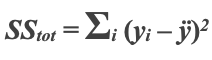The formula for sum of totals (with y being an actual value and ÿ being the moving average of these values),

###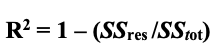And finally, that for the coefficient itself also referred to as R squared.

What this coefficient does is measure the extent to which our xs are influencing the y. This is important because, as mentioned, there are periods when regression ebbs meaning it is safer to stay away from the markets. By monitoring this through a filter we are more likely to trade when the system is dependable. Typically, you want this coefficient to be above 0 with 1 being the ideal. The input parameter used in defining our threshold will be 'm_open_determination’ or ‘m_close_determination’, once again subject to number of open positions. If the coefficient of determination as computed by the 'CheckDetermination' function, listed below, is less than this parameter then the long or short conditions will return zero.

```//+------------------------------------------------------------------+
//|                                                                  |
//+------------------------------------------------------------------+
int CSignalDUAL_RA::CheckDetermination(int ind,bool close)
{
int _check=0;
m_h_ma.Refresh(-1);m_l_ma.Refresh(-1);
double _det=0.0,_ss_res=0.0,_ss_tot=0.0;
for(int r=0;r<m_size;r++)
{
_ss_res+=pow(Data(r,close)-GetY(r+1,close),2.0);
_ss_tot+=pow(Data(r,close)-((m_l_ma.Main(r)-m_l_ma.Main(r+1))-(m_h_ma.Main(r)-m_h_ma.Main(r+1))),2.0);
}

if(_ss_tot!=0.0)
{
_det=(1.0-(_ss_res/_ss_tot));
if(_det>=m_open_determination)
{
double _threshold=0.0;
for(int r=0; r<m_size; r++){ _threshold=fmax(_threshold,fabs(Data(r,close))); }

double _y=CheckCollinearity(ind,close);

_check=int(round(100.0*_y/fmax(fabs(_y),fabs(_threshold))));
}
}
//---
return(_check);
}```

Once we can check for the coefficient of determination we would have a workable signal. What follows next would be assembling this signal in the MQL5 wizard into an expert advisor.

### 3.  Assembling with MQL5 Wizard

3.1  Custom ancillary code listing can be used together with the code from the MQL5 wizard in assembling an expert advisor. This is entirely optional and is to the style of the trader. For the purposes of this article we are going to look at custom pending order opening that is based on the symbol’s prevailing ATR, as well as a system of trailing open positions that is based on the same indicator. We will not use take profit targets.

3.1.1  ATR based pending orders can be set by overloading the functions ‘OpenLongParams’ and ‘OpenShortParams’ and customizing them in our signal class as shown below.

```//+------------------------------------------------------------------+
//| Detecting the levels for buying                                  |
//+------------------------------------------------------------------+
bool CSignalDUAL_RA::OpenLongParams(double &price,double &sl,double &tp,datetime &expiration)
{
CExpertSignal *general=(m_general!=-1) ? m_filters.At(m_general) : NULL;
//---
if(general==NULL)
{
m_ATR.Refresh(-1);
//--- if a base price is not specified explicitly, take the current market price
double base_price=(m_base_price==0.0) ? m_symbol.Ask() : m_base_price;

//--- price overload that sets entry price to be based on ATR
price      =m_symbol.NormalizePrice(base_price-(m_price_level*(m_ATR.Main(0)/m_symbol.Point()))*PriceLevelUnit());

sl         =0.0;
tp         =0.0;
expiration+=m_expiration*PeriodSeconds(m_period);
return(true);
}
//---
return(general.OpenLongParams(price,sl,tp,expiration));
}
//+------------------------------------------------------------------+
//| Detecting the levels for selling                                 |
//+------------------------------------------------------------------+
bool CSignalDUAL_RA::OpenShortParams(double &price,double &sl,double &tp,datetime &expiration)
{
CExpertSignal *general=(m_general!=-1) ? m_filters.At(m_general) : NULL;
//---
if(general==NULL)
{
m_ATR.Refresh(-1);
//--- if a base price is not specified explicitly, take the current market price
double base_price=(m_base_price==0.0) ? m_symbol.Bid() : m_base_price;

//--- price overload that sets entry price to be based on ATR
price      =m_symbol.NormalizePrice(base_price+(m_price_level*(m_ATR.Main(0)/m_symbol.Point()))*PriceLevelUnit());

sl         =0.0;
tp         =0.0;
expiration+=m_expiration*PeriodSeconds(m_period);
return(true);
}
//---
return(general.OpenShortParams(price,sl,tp,expiration));
}```

The MQL5 wizard generated expert advisor has an input parameter ‘Signal_PriceLevel’. By default, it is zero but if assigned a value it represents the distance, in price points of the traded symbol, from the current price at which a market order will be placed. When this input is negative stop orders are placed. When positive limit orders are placed. It is a double data type. For our purposes this input will be a fraction or multiple of the current price points in the ATR.

3.1.2   ATR trailing class is also a customised ‘CExpertTrailing’ class that also uses the ATR to set and move the stop loss. The implementation of its key functions is in the listing below.

```//+------------------------------------------------------------------+
//| Checking trailing stop and/or profit for long position.          |
//+------------------------------------------------------------------+
bool CTrailingATR::CheckTrailingStopLong(CPositionInfo *position,double &sl,double &tp)
{
//--- check
if(position==NULL)
return(false);
//---
m_ATR.Refresh(-1);
double level =NormalizeDouble(m_symbol.Bid()-m_symbol.StopsLevel()*m_symbol.Point(),m_symbol.Digits());

//--- sl adjustment to be based on ATR
double new_sl=NormalizeDouble(level-(m_atr_weight*(m_ATR.Main(0)/m_symbol.Point())),m_symbol.Digits());

double pos_sl=position.StopLoss();
double base  =(pos_sl==0.0) ? position.PriceOpen() : pos_sl;
//---
sl=EMPTY_VALUE;
tp=EMPTY_VALUE;
if(new_sl>base && new_sl<level)
sl=new_sl;
//---
return(sl!=EMPTY_VALUE);
}
//+------------------------------------------------------------------+
//| Checking trailing stop and/or profit for short position.         |
//+------------------------------------------------------------------+
bool CTrailingATR::CheckTrailingStopShort(CPositionInfo *position,double &sl,double &tp)
{
//--- check
if(position==NULL)
return(false);
//---
m_ATR.Refresh(-1);

//--- sl adjustment to be based on ATR
double new_sl=NormalizeDouble(level+(m_atr_weight*(m_ATR.Main(0)/m_symbol.Point())),m_symbol.Digits());

double pos_sl=position.StopLoss();
double base  =(pos_sl==0.0) ? position.PriceOpen() : pos_sl;
//---
sl=EMPTY_VALUE;
tp=EMPTY_VALUE;
if(new_sl<base && new_sl>level)
sl=new_sl;
//---
return(sl!=EMPTY_VALUE);
}```

Once again the 'm_atr_weight' will be an optimisable parameter, like with 'm_price_level', that sets how close we can trail open positions.

3.2  Wizard assembly will then be done in straightforward fashion with the only notable stages being the selection of our signal as shown below.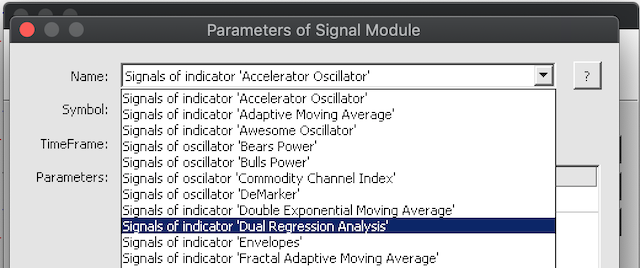And the addition of our custom trailing method shown below.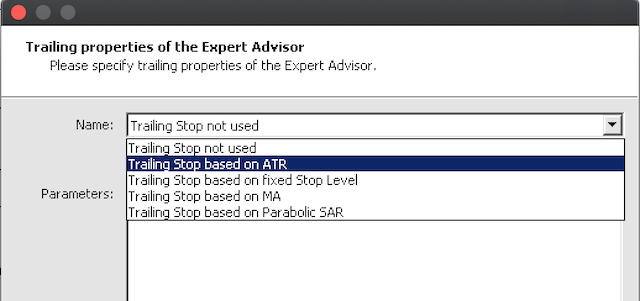### 4. Testing in Strategy Tester

4.1  Compilation is what would follow assembly in the MQL5 wizard in order to create the expert advisor file and also confirm that there are no errors in our code.

4.2  Default inputs of the expert advisor would also need to be set in the strategy tester inputs tab. The key here is to ensure ‘Signal_TakeLevel’ and ‘Signal_StopLevel’ are set to zero. This is because as mentioned, for the purpose of this article, the exit is defined only by the trailing stop or the ‘Signal_ThresholdClose’ input parameter.

4.3  Optimisation should be performed ideally with the real ticks of the broker you intend to trade with. For this article we will optimize EURUSD on the 4-hour timeframe across its V shaped period of 2018.01.01 to 2021.01.01. For comparison purposes we will run two optimizations: the first one will only use market orders, while the second will be open to pending orders. I use ‘open to’ pending orders because we will still consider the option of using only market orders given that ‘Signal_PriceLevel’ can be zero since optimization is from a negative value to a positive value. Optimization can be set up prior for the option that uses pending orders as shown below. The only difference between this and the option that does not use pending orders is the later will leave 'Signal_PriceLevel' input parameter at 0 not part of the optimised inputs.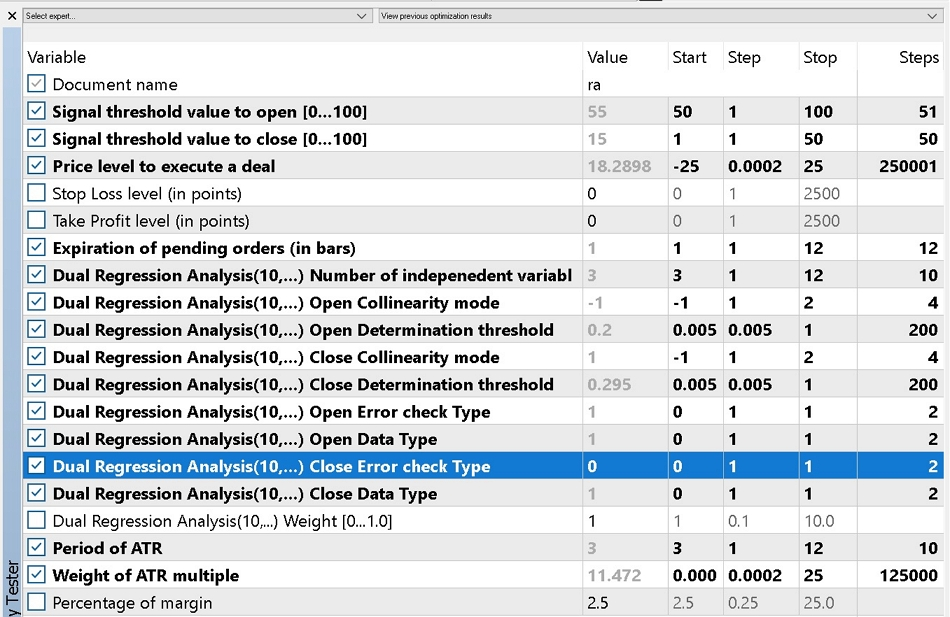4.4  Results from our optimisation are presented below. First is the report and equity curve of the best results from trading only with market orders.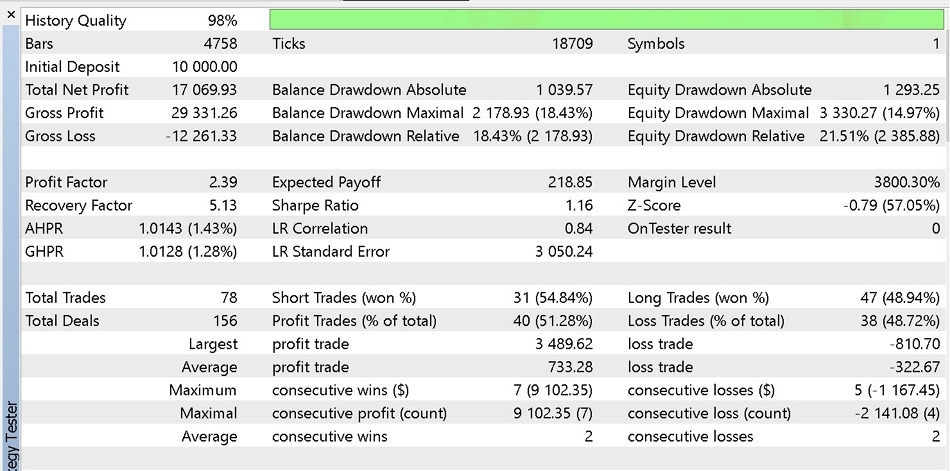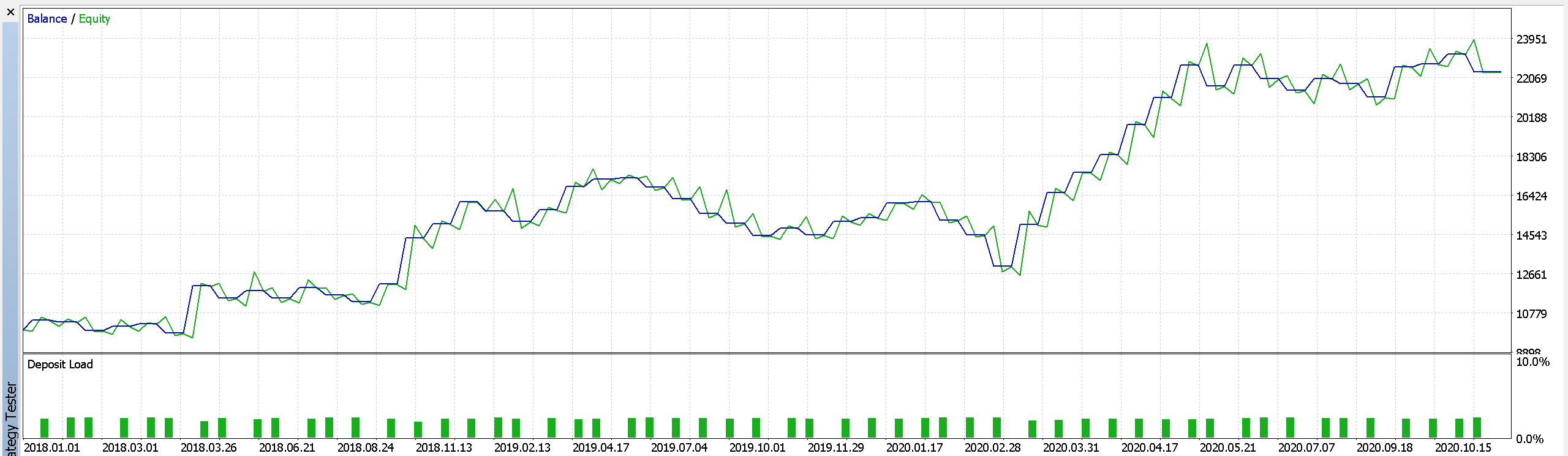Part of report 1,

Then a likewise report and curve from using pending orders.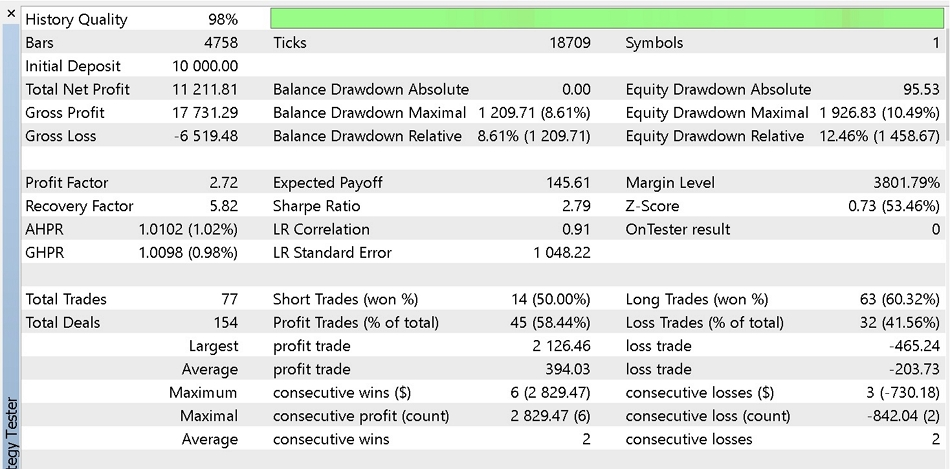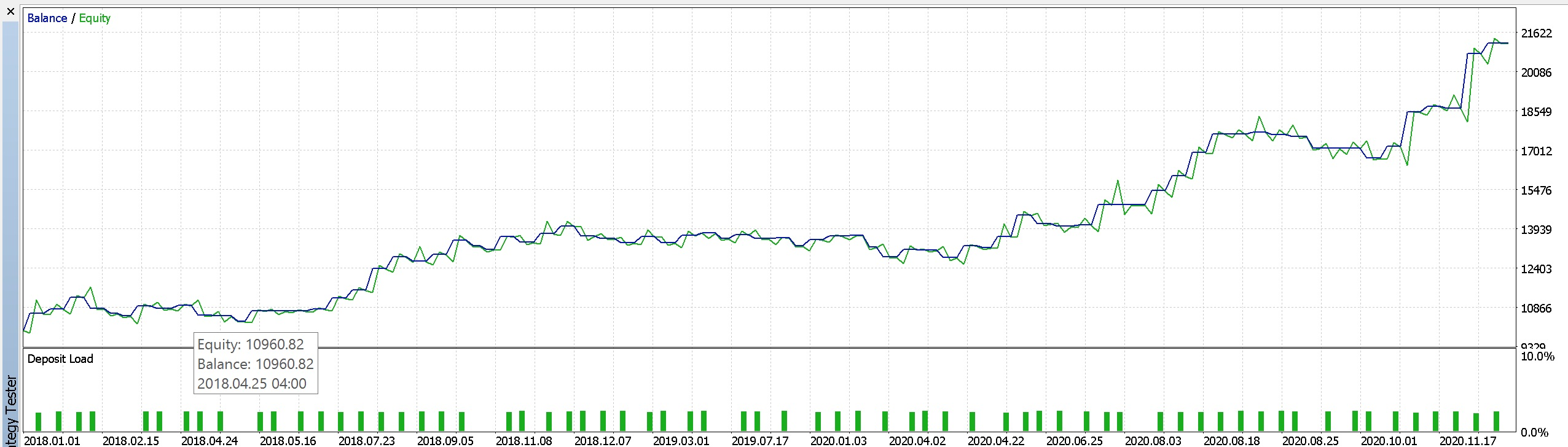Part of report 2.

It appears our regression analysis signal benefits from using pending orders by having less drawdowns at the sacrifice of some profits. Other modifications could be made as well to enhance this system such as changing the trailing stop class, or the money management type. For our testing purposes we used fixed margin percent and we optimised with our criteria set to 'complex criterion'. It is desirable however to test as extensively as possible on historical tick data and do sufficient forward walks before deploying things which are beyond this article's scope.

### 5. Conclusion

Attached files |
TrailingATR.mqh (6.24 KB)
SignalDUAL_RA.mqh (18.75 KB)

#### Other articles by this author

Last comments | Go to discussion (1)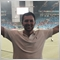| 9 Sep 2022 at 16:49

Hi Stephen,

Very good article. I made some tests and it returned good results. Looks like we have an encouraging trade system.

I would like to know how can I use input with non-fixed timeframes for optimization.

I've tried to change these lines, but OnInit "returned non-zero code 1" with no error message.

```//--- inputs for expert
input string Expert_Title                     ="Regr2"; // Document name
ulong        Expert_MagicNumber               =26034;   //
bool         Expert_EveryTick                 =false;   //
input ENUM_TIMEFRAMES   timeframe             =PERIOD_M5;      //TimeFrame
//--- inputs for main signal
.
.
.
.
int OnInit()
{
//--- Initializing expert
if(!ExtExpert.Init(Symbol(),timeframe,Expert_EveryTick,Expert_MagicNumber))
{
//--- failed
printf(__FUNCTION__+": error initializing expert");
ExtExpert.Deinit();
return(INIT_FAILED);
}
//--- Creating signal
.
.
.
.
```

Can you help me?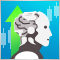How to master Machine Learning
Check out this selection of useful materials which can assist traders in improving their algorithmic trading knowledge. The era of simple algorithms is passing, and it is becoming harder to succeed without the use of Machine Learning techniques and Neural Networks.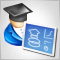Developing a trading Expert Advisor from scratch (Part 8): A conceptual leap
What is the easiest way to implement new functionality? In this article, we will take one step back and then two steps forward.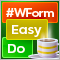DoEasy. Controls (Part 4): Panel control, Padding and Dock parameters
In this article, I will implement handling Padding (internal indents/margin on all sides of an element) and Dock parameters (the way an object is located inside its container).DoEasy. Controls (Part 3): Creating bound controls
In this article, I will create subordinate controls bound to the base element. The development will be performed using the base control functionality. In addition, I will tinker with the graphical element shadow object a bit since it still suffers from some logic errors when applied to any of the objects capable of having a shadow.